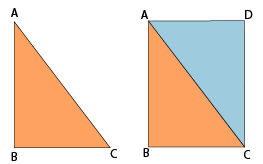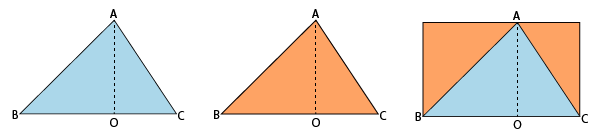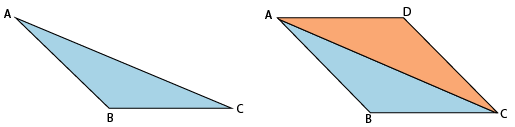Area of triangle

## Materials required for real lab:

Chart paper, pencil, compass, scale, a pair of scissors, cello tape.

## Procedure for Right angled triangle:

### As performed in real lab:

1. Cut a right angle triangle.
2. Cut a triangle congruent to the right angle triangle.
3. Align the hypotenuse of the two triangles to obtain a rectangle.[Fig(A)]### As performed in the simulator:

1. Create a ▲ ABC by providing its three sides. For a right angled triangle with sides a, b, c where c hypotenuse, then a2 + b2 = c2.
2. Click on ▲ ABC to create its replica.
3. Place replicated triangle such that hypotenuse of both triangle will cover each other. Use button 'clockwise' to rotate triangle clockwise. Use button 'Anticlockwise' to rotate triangle Anticlockwise.
4. See the observation.

### Observation:

We can observe that two congruent triangles aligned on hypotenuse forms a rectangle.
∴  Area of □ ABCD = 2 x Area of ▲ ABC
∴ Area of ▲ ABC = 1/2 x Area of □ ABCD
= 1/2 x [base of □ ABCD X height of □ ABCD]
= 1/2 x [BC x AB]
= 1/2 x base of triangle ABC x height of triangle ABC=1/2 x base x height

## Procedure for Acute Angled Triangle:

### As performed in the real lab:

1. Cut an acute angle triangle and draw the perpendicular from the vertex to the opposite side.
2. Cut a triangle congruent to it and cut this triangle along perpendicular.
3. Align the hypotenuse of these cut outs to the given triangle in order to obtain a rectangle.[Fig(B)]### As performed in simulator:

1. Create a ▲ ABC by providing its three sides. Triangle is Acute angled triangle if its square of longest side is less than to sum of squares of other two sides.
2. Next step is to draw perpendicular from A To line BC.
• Click on SetSquare in the 'Tools' to use it.
• Drag this set square and place at position such that point A will perpendicular to base BC.
3. Next step is to create two replica triangles of ▲ ABO and ▲ AOC respectively. Click on 'Cut Triangle' button to create these replicas.
4. Next step is to place these colored triangles at appropriate positions.
• First you  have to place yellow colored triangle and then red colored triangle.
• Drag yellow colored triangle and place along with its hypotenuse to side AB of ▲ AOB which finally forms a rectangle AOBD.
• Drag Red colored triangle and place along with its hypotenuse to side AC of ▲ AOC which finally forms a rectangle AOCE.
• You can use 'clockwise' button to rotate triangle clockwise.
• You can use 'Anti-clockwise' button to rotate triangle Anti-clockwise.
5. See the observation.

### Observation:

As □ DBCE is formed with ▲ ABC and 2 congruent triangles ABO and AOC.
∴  Area of □  DBCE =  Area of ▲ ABC + (Area of ▲ ABO + Area of ▲ AOC)
=  Area of ▲ ABC + Area of ▲ ABC
= 2 x Area of ▲ ABC
Area of ▲ ABC = 1/2 x Area of □ DBCE
= 1/2 x [base of □ DBCE X height of □ DBCE]
= 1/2 x [BC x DB] = 1/2 x [BC x AO]
= 1/2 x base of triangle ABC x height of triangle ABC = 1/2 x base x height

## For Obtuse Angle Triangle:

### As performed in the real lab:

1. Cut an obtuse angle triangle.
2. Cut a triangle congruent to this obtuse angle triangle.
3. Align the greatest side of the two triangles in order to obtain parallelogram.[Fig(C)]### As performed in simulator:

1.     Create a ▲ ABC by providing its three side.
2.     Triangle is Obtuse angle triangle  if its  square of longest side is greater than to sum of squares of other two sides.
3.     Click on ▲ ABC to create its replica.
4.     Place this replicated triangle such that it will forms parallelogram.
5.     Use button 'clockwise' to rotate triangle clockwise.
6.     Use button 'Anticlockwise' to rotate triangle Anticlockwise.
7.     Place replicated triangle such that hypotenuse of both triangle will cover each other.
8.     See the observation.

### Observation:

You can observe that aligning these two congruent triangles forms a parallelogram.

As per property of parallelogram ▲ ABC and ▲ ADC are congruent

∴ Area of ▱ ABCD = Area of ▲ ABC + Area of ▲ ADC
= 2 x area of ▲ ABC
∴Area of ▲ ABC  = 1/2 x Area of ▱ ABCD
=  1/2 x [base of ▱ ABCD X height of ▱ ABCD]
= 1/2 x [BC x height of ▲ ABC]
= 1/2 x base of ▲ ABC x height of ▲ ABC = 1/2 x base x height